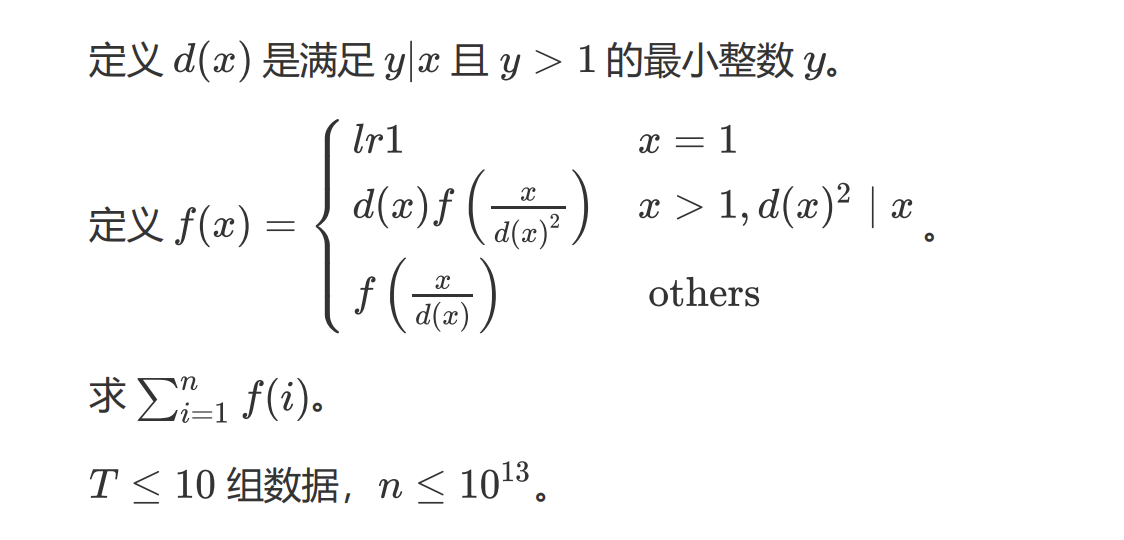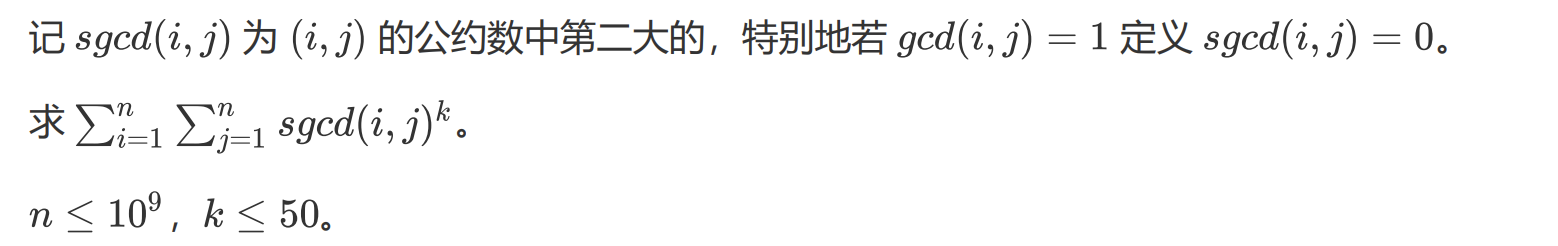2.18 数论题

## T1

$T\le 5000,a,b\le 10^9$ 求：

## T2

$1 \le a \le b \le 10^{10}$ 求：

## T3

$n \le 10^{12}$ 求：

## T4$g(x)$ 是 $x$ 的最大平方因子开根后的结果。

## T5## T7

$T \le 20 , x,a,b \le 10^9$